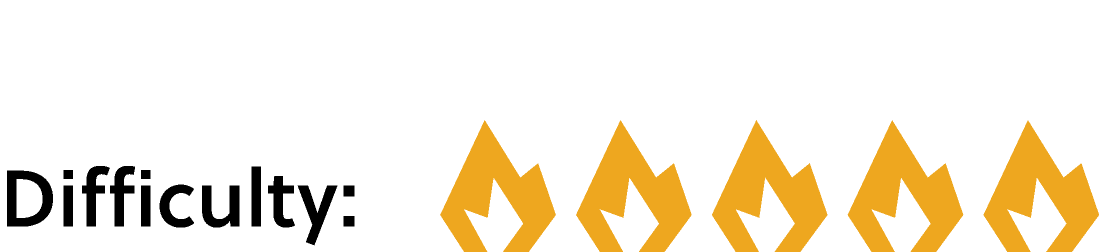## Fill the Pinwheel

Grid Fill puzzles like the ones below are similar to Sudoku puzzles in that they ask you to place numbers in squares in a certain way, but the similarities end there.

If you're familiar with how these work, jump straight to today's challenge. If you want to look through some worked examples, though, keep reading.

One of the differences between these puzzles and Sudoku is that a Grid Fill solution can have a number appearing more than once in the same row or column. The goal is to drag number tiles from the bank to squares on the grid so the rows and columns sum to the indicated values.

For example, a solution to the puzzle below will have numbers in the top row with a sum of $3.$ Note that the middle square of that row is gray and will not accept a number tile, so its effective value is zero. By clicking the right arrow below the puzzle, you can view a demonstration of a puzzle solution complete with the underlying logic. If you need to see a step again, click the left arrow.

The puzzle below is a bit different; there is an inexhaustible source of $0$ tiles, so every time you take a $0$ tile from the bank and place it on the grid, the tile will be replaced with a new one. The other tiles, however, can be used up. There are three $1$ tiles available, but only one $5$ tile. Again, use the arrows below the puzzle to see solution steps.

# Today's Challenge

How many solutions does this puzzle have?

Hint: Use the symmetries of the grid. $\\[1em]$